Function Repository Resource:

# StudentTValue

Calculate the Student's t-value that corrects for the bias of the unknown standard deviation in the statistical uncertainty of a measurement

Contributed by: Julien Kluge
 ResourceFunction["StudentTValue"][ν] calculates the Student's t-value for a StudentTDistribution with ν degrees of freedom. ResourceFunction["StudentTValue"][ν,Around[value,error]] returns the Around expression with error scaled by Student’s t with ν degrees of freedom. ResourceFunction["StudentTValue"][ν,{Around[v1,e1],Around[v2,e2],…}] returns a list of Around expressions. ResourceFunction["StudentTValue"][{v1,v2,…}] returns an Around created as by MeanAround with an error scaled by the Student’s t-value.

## Details and Options

The degrees of freedom parameter ν must be a positive integer.
Use of this function is recommeded when a statistical measurement has less than 20 data points.
ResourceFunction["StudentTValue"] takes the option ConfidenceLevel.

## Examples

### Basic Examples (3)

Calculate the Student’s t-value for two degrees of freedom:

 In:=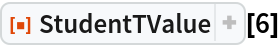Out=Scale the error of an around by the Student’s t-value:

 In:=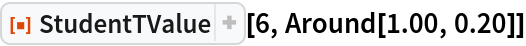Out=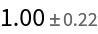Calculate the value of a measurement with a Student’s t-value scaled error:

 In:=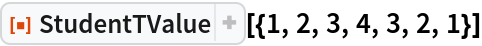Out=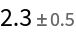### Scope (3)

The function can return an exact value in the single argument form. Use N to get a numerical value:

 In:=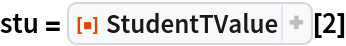Out=In:=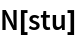Out=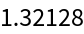The function can handle a list of Around values:

 In:=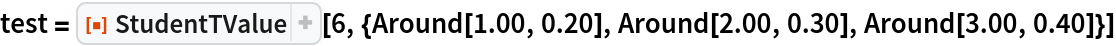Out=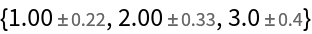StudentTValue can handle asymmetric Around:

 In:=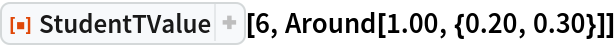Out=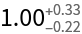### Options (1)

StudentTValue will calculate the Student’s t-value according to the specified ConfidenceLevel (which is per default at 0.683=1σ):

 In:=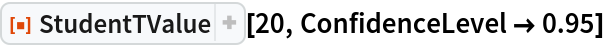Out=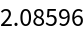### Applications (1)

Calculate the statistical uncertainty for measurements with only a few data points:

 In:=Out=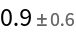### Properties and Relations (2)

The Student’s t-value for a List is equal to the Student’s t-value of the resulting MeanAround of the data with the degrees of freedom equal to the number of data points minus one:

 In:=Out=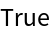For many measurements, StudentTValue will tend towards one (if the ConfidenceLevel option is set to Automatic as default):

 In:=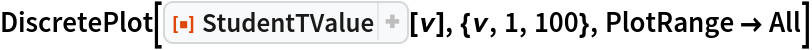Out=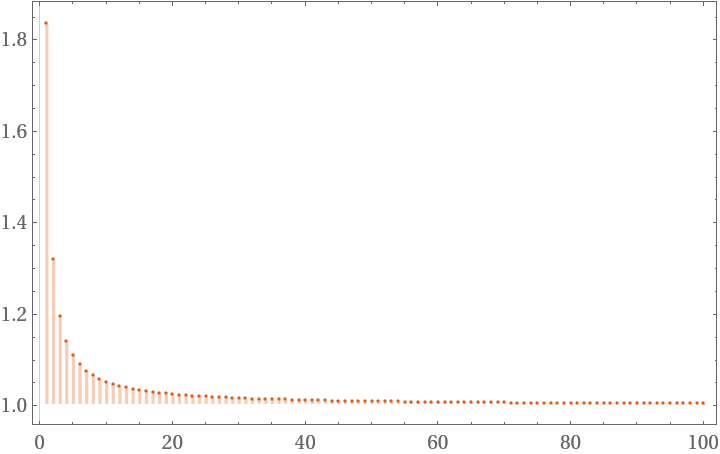### Possible Issues (2)

The function only accepts integers as the degree of freedom parameter ν:

 In:=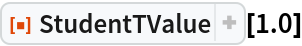Out=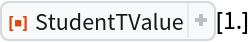The function returns an infinitely-big error interval for just one value:

 In:=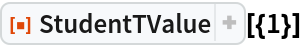Out=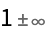### Neat Examples (1)

The error interval from StudentTValue always encloses the one from MeanAround but converges for a high enough number of measurements:

 In:=Out=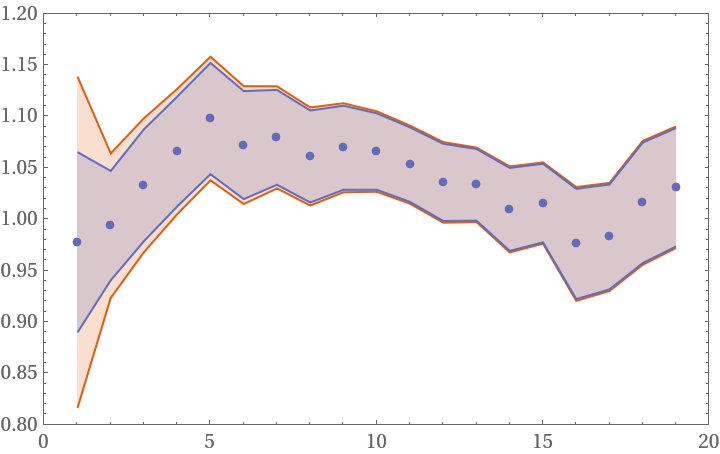Julien Kluge

## Version History

• 1.0.0 – 21 April 2020

## Author Notes

This function could be built into MeanAround as an option.
Julien Kluge Quantum Optical Metrology; Joint Lab Integrated Quantum Sensors Department of Physics Humboldt-Universität zu Berlin julien@physik.hu-berlin.de